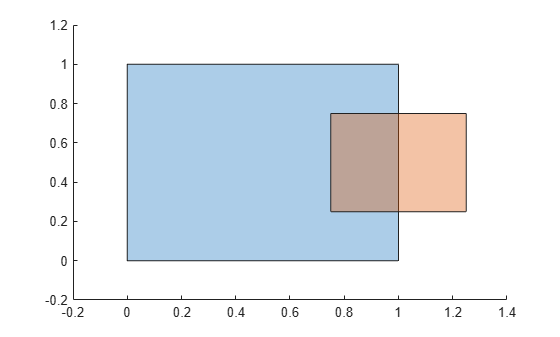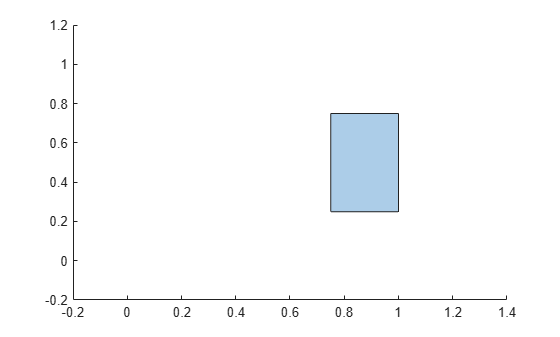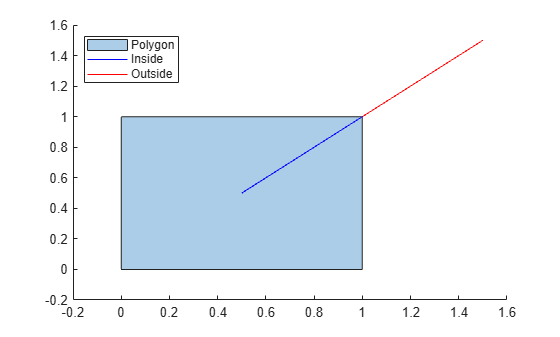# intersect

Intersection of `polyshape` objects

## Syntax

``polyout = intersect(poly1,poly2)``
``polyout = intersect(polyvec)``
``[polyout,shapeID,vertexID] = intersect(poly1,poly2)``
``[polyout,shapeID,vertexID] = intersect(polyvec)``
``___ = intersect(___,'KeepCollinearPoints',TF)``
``[in,out] = intersect(poly1,lineseg)``

## Description

example

````polyout = intersect(poly1,poly2)` returns a `polyshape` object whose regions are the geometric intersection of two `polyshape` objects. The intersection contains the regions of `poly1` and `poly2` that overlap. `poly1` and `poly2` must have compatible array sizes.```

example

````polyout = intersect(polyvec)` returns a `polyshape` object whose regions are the intersection of all the `polyshape` objects in the vector `polyvec`. The intersection contains the regions where all the `polyshape` objects in `polyvec` overlap.```

example

````[polyout,shapeID,vertexID] = intersect(poly1,poly2)` also returns vertex mapping information from the vertices in `polyout` to the vertices in `poly1` and `poly2`. The `intersect` function only supports this syntax when `poly1` and `poly2` are scalar `polyshape` objects.The `shapeID` elements identify whether the corresponding vertex in `polyout` originated in `poly1`, `poly2`, or was created from the intersection. `vertexID` maps the vertices of `polyout` to the vertices of `poly1`, `poly2`, or the intersection.```
````[polyout,shapeID,vertexID] = intersect(polyvec)` returns vertex mapping information from `polyout` to each element of the vector of `polyshape` objects `polyvec`.```
````___ = intersect(___,'KeepCollinearPoints',TF)` specifies whether to keep or remove collinear points in `polyout` for any of the previous syntaxes.```

example

````[in,out] = intersect(poly1,lineseg)` returns the line segments of `lineseg` that are inside and outside of `poly1`. The matrix `lineseg` has two columns. The first column defines the x-coordinates of the line segments and the second column defines the corresponding y-coordinates.`intersect` supports this syntax only when `poly1` is a scalar `polyshape` and `lineseg` contains no self-intersections.```

## Examples

collapse all

Create and plot two polygons.

```poly1 = polyshape([0 0 1 1],[1 0 0 1]); poly2 = polyshape([0.75 1.25 1.25 0.75],[0.25 0.25 0.75 0.75]); plot(poly1) hold on plot(poly2)````figure`

Compute and plot the intersection of `poly1` and `poly2`.

`polyout = intersect(poly1,poly2)`
```polyout = polyshape with properties: Vertices: [4x2 double] NumRegions: 1 NumHoles: 0 ```
```plot(polyout) xlim([-0.2 1.4]); ylim([-0.2 1.2]);```Create a vector containing two polygons.

```polyarray1 = polyshape([0 0 1 1],[1 0 0 1]); polyarray2 = polyshape([0.75 1.25 1.25 0.75],[0.25 0.25 0.75 0.75]); poly1 = [polyarray1 polyarray2]```
```poly1 = 1x2 polyshape array with properties: Vertices NumRegions NumHoles ```
```plot(poly1(1)) hold on plot(poly1(2))````figure`

Compute the intersection of the elements of `poly1`.

`polyout = intersect(poly1)`
```polyout = polyshape with properties: Vertices: [4x2 double] NumRegions: 1 NumHoles: 0 ```
```plot(polyout) xlim([-0.2 1.4]); ylim([-0.2 1.2]);```Create two polygons and compute their intersection. Display the vertex coordinates of the intersection and the corresponding vertex mapping information.

```poly1 = polyshape([0 0 1 1],[1 0 0 1]); poly2 = polyshape([0.75 1.25 1.25 0.75],[0.25 0.25 0.75 0.75]); [polyout,shapeID,vertexID] = intersect(poly1,poly2); [polyout.Vertices shapeID vertexID]```
```ans = 4×4 0.7500 0.2500 2.0000 1.0000 0.7500 0.7500 2.0000 2.0000 1.0000 0.7500 0 0 1.0000 0.2500 0 0 ```

The first two vertices of the intersection originated in `poly2`, since the corresponding values in `shapeID` are 2.These vertices are the first and second vertices in the property `poly2.Vertices`, respectively, since their corresponding values in `vertexID` are 1 and 2. The last two vertices of `polyout` were created from the intersection because the corresponding values in `shapeID` and `vertexID` are 0.

Create a rectangular polygon and a line segment.

```poly1 = polyshape([0 0 1 1],[1 0 0 1]); lineseg = [0.5 0.5; 1.5 1.5];```

Compute the intersection of the polygon with the line segment, and determine which sections of the line segment are inside or outside of the polygon.

```[in,out] = intersect(poly1,lineseg); plot(poly1) hold on plot(in(:,1),in(:,2),'b',out(:,1),out(:,2),'r') legend('Polygon','Inside','Outside','Location','NorthWest')```## Input Arguments

collapse all

First input `polyshape`, specified as a scalar, vector, matrix, or multidimensional array.

Data Types: `polyshape`

Second input `polyshape`, specified as a scalar, vector, matrix, or multidimensional array.

Data Types: `polyshape`

`polyshape` vector.

Data Types: `polyshape`

Line segment coordinates, specified as a two-column matrix. The first column defines the x-coordinates of the line segments and the second column defines the y-coordinates. `lineseg` must have at least two rows and contain no self-intersections.

Data Types: `double` | `single` | `int8` | `int16` | `int32` | `int64` | `uint8` | `uint16` | `uint32` | `uint64`

Collinear vertices indicator, specified as `false` or `true`:

• `false` — Remove collinear points so that the output `polyshape` contains the fewest vertices necessary to define the boundaries.

• `true` — Keep all collinear points as vertices.

When the `'KeepCollinearPoints'` parameter is not specified, its value is assigned according to the values used when creating the input `polyshape` objects:

• If the value was `true` for each input `polyshape` when they were created, then the value for the output `polyshape` is set to `true`.

• If the value was `false` for each input `polyshape` when they were created, then the value for the output `polyshape` is set to `false`.

• If the values for the input `polyshape` objects do not match, then the value for the output `polyshape` is set to `false`.

Data Types: `logical`

## Output Arguments

collapse all

Output `polyshape`, returned as a scalar, vector, matrix, or multidimensional array.

• If you input two `polyshape` arguments, then they must have compatible sizes. For example, if two input `polyshape` vectors have different lengths M and N, then they must have different orientations (one must be a row vector and one must be a column vector). `polyout` is then M-by-N or N-by-M depending on the orientation of each input vector. For more information on compatible array sizes, see Compatible Array Sizes for Basic Operations.

• If you provide a single input argument `polyvec`, then `polyout` is a scalar `polyshape` object.

Shape ID, returned as a column vector whose elements each represent the origin of a vertex in the intersection.

• The length of `shapeID` is equal to the number of rows in the `Vertices` property of the output `polyshape`.

• The elements of `shapeID` depend on the number of input arguments:

• If you provide two input arguments `poly1` and `poly2`, then they must be scalar `polyshape` objects. The value of an element in `shapeID` is 0 when the corresponding vertex of the output `polyshape` was created by the intersection. An element is 1 when the corresponding vertex originated from `poly1`, and 2 when it originated from `poly2`.

• If you provide one input argument `polyvec` that is a vector of `polyshape` objects, then `shapeID` contains the element index of `polyvec` from which the corresponding output vertex originated. The value of an element is 0 when the corresponding vertex was created by the intersection.

Data Types: `double`

Vertex ID, returned as a column vector whose elements map the vertices in the output `polyshape` to the vertices in the `polyshape` of origin. The elements of `vertexID` contain the row numbers of the corresponding vertices in the `Vertices` property of the input `polyshape`. An element is 0 when the corresponding vertex of the output `polyshape` was created by the intersection.

The length of `vertexID` is equal to the number of rows in the `Vertices` property of the output `polyshape`. If you provide two input `polyshape` objects, then `intersect` only supports this output argument if they are scalar.

Data Types: `double`

Inside line segment coordinates, returned as a two-column matrix. The first column of `in` contains the x-coordinates of the line segments inside the input `polyshape`, and the second column contains the corresponding y-coordinates.

Data Types: `double`

Outside line segment coordinates, returned as a two-column matrix. The first column of `out` contains the x-coordinates of the line segments outside of the input `polyshape`, and the second column contains the corresponding y-coordinates.

Data Types: `double`